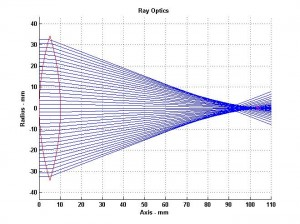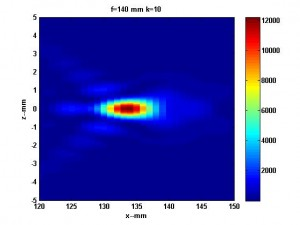# Optical Applications of Full Diffraction

Revised May 30, 2011

Earlier blogs on this topic discussed the relation of  The Theory of Scintillation with Applications to Remote Sensing, which uses the forward propagation method (FPE) , to optical methods based on the Huygens-Fresnel construction.  The revised paper linked below presents a more cohesive development.  There is a regime where wavelength does not dictate sampling requirements.  In this common regime, identical computational methods can be used for optics, acoustics, and radio frequency applications.  This regime includes the Fraunhofer diffraction limit.  However, the FPE is formally unconstrained.

A case in point is ray optics, which is based on a wavelength independent geometrical construction.  An example is shown in the Figure below.  The problem is the omission of diffraction.The paper uses Fourier domain methods to compute the evolution of the wavefront from the aperture stop where ray optics gives an accurate representation to the focal plane.  The problem is that full diffraction computation requires wavelength scale sampling.

The figure below shows an example of Fraunhofer diffraction where computation at optical wavelengths is easily achieved.The two figures below show the development of a focus at mm wavelengths where full-diffraction computations are feasible.  The details are discussed in the unpublished paper submitted for publication in Antennas & Propagation Magazine linked below.